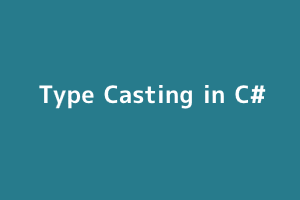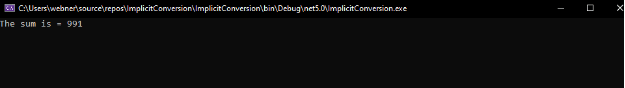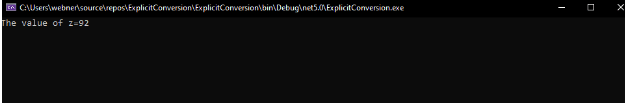Author - StudySection Post Views - 60 views# Type Casting in C#

Conversion of the data type of a variable to another type is called Type Casting. After the declaration of a variable, we cannot declare it again. The value of a variable cannot be assigned to another type of variable until we change its type.

Suppose we have a variable whose type is an integer and we need to pass it to a method parameter whose type is double. Such operations are called Type Conversion.

We have different types of conversions in C# i.e.,

• ## IMPLICIT CONVERSION:

In this type of conversion, there is no loss of data. In this, the variables with smaller data types are converted to larger data types implicitly.
Let’s look at an example of Implicit type conversion
```using System; namespace ImplicitConversion { class Program { static void Main(string[] args) { int x = 530; int y = 461; long sum; sum = x + y; Console.WriteLine("The sum is = " + sum); Console.ReadLine(); } } }```
OUTPUTIn this example, we have two variables of type integer i.e., x and y and we store the value of the sum of the two in a variable sum which is of type long. In this case, our output comes out to be fine as the data type integer gets implicitly converted to the larger data type i.e., long.

• ### EXPLICIT CONVERSION

This type of conversion is done when there is a loss of data. This is done with the help of the type cast operator(). This is done in case when we have to convert the larger data type to a smaller one.

Let’s look at an example of an Explicit Type of Conversion:
```using System; using System.Collections.Generic; using System.Linq; using System.Text; using System.Threading.Tasks; namespace ExplicitConversion { class Program { static void Main(string[] args) { double d = 92.75; int z; // here we do the cast double to int. z = (int)d; Console.WriteLine("The value of z=" + z); Console.ReadKey(); } } } ```

OUTPUTHere in the example the variable d which is of long data type is converted to an integer and is rounded off to the closest figure.

Knowledge of .NET is quite rewarding in the IT industry. If you have got some skills in the .NET framework then a .NET certification from StudySection can prove to be a good attachment with your resume. You can go for a foundation level certificate as well as an advanced level certificate in the .NET framework.